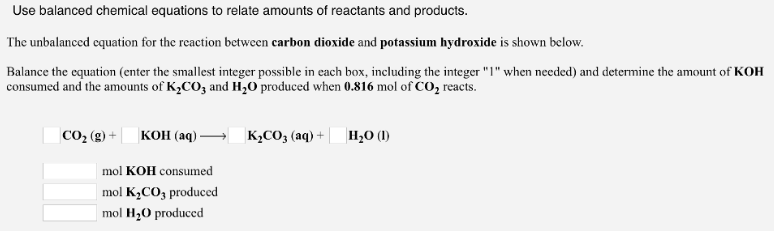# Problem: Use balanced chemical equations to relate amounts of reactants and products. The unbalanced equation for the reaction between carbon dioxide and potassium hydroxide is shown below. Balance the equation (enter the smallest integer possible in each box, including the integer "1" when needed) and determine the amount of KOH consumed and the amounts of K2CO3 and H2O produced when 0.816 mol of CO2 reacts. _CO2 (g) + _KOH (aq) → _K2CO3 (aq) + _H2O (l) mol

###### FREE Expert Solution
94% (32 ratings)###### Problem Details

Use balanced chemical equations to relate amounts of reactants and products.

The unbalanced equation for the reaction between carbon dioxide and potassium hydroxide is shown below.

Balance the equation (enter the smallest integer possible in each box, including the integer "1" when needed) and determine the amount of KOH consumed and the amounts of K2CO3 and H2O produced when 0.816 mol of CO2 reacts.

_CO2 (g) + _KOH (aq) → _K2CO(aq) + _H2O (l) mol